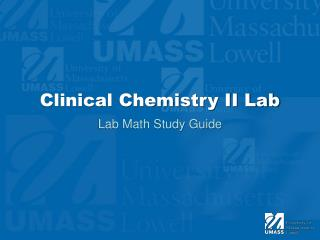Download PresentationClinical Chemistry II Lab

# Clinical Chemistry II Lab - PowerPoint PPT Presentation

Clinical Chemistry II Lab. Lab Math Study Guide. Anion Gap Calculation. Na + + K + = Total cations Cl - + HCO 3 - = Total anions Total cations – Total anions = Anion gap. Practice AG Calculation. Sodium = 140 mmol/L Potassium = 4.9 mmol/L Chloride = 109 mmol/L Bicarbonate = 24 mmol/L.I am the owner, or an agent authorized to act on behalf of the owner, of the copyrighted work described.
Download Presentation## Clinical Chemistry II Lab

Download Policy: Content on the Website is provided to you AS IS for your information and personal use and may not be sold / licensed / shared on other websites without getting consent from its author.While downloading, if for some reason you are not able to download a presentation, the publisher may have deleted the file from their server.

- - - - - - - - - - - - - - - - - - - - - - - - - - E N D - - - - - - - - - - - - - - - - - - - - - - - - - -
Presentation Transcript
1. Clinical Chemistry II Lab Lab Math Study Guide

2. Anion Gap Calculation • Na+ + K+ = Total cations • Cl- + HCO3- = Total anions • Total cations – Total anions = Anion gap

3. Practice AG Calculation • Sodium = 140 mmol/L • Potassium = 4.9 mmol/L • Chloride = 109 mmol/L • Bicarbonate = 24 mmol/L Total cations = 145 mmol/L Total anions = 133 mmol/L Anion Gap = 12 mmol/L

4. Molarity • Main equation grams = (molar mass) x (molarity) x (volume) Units molar mass = g/mole molarity = moles/L volume = liters

5. Molarity • Secondary Equation grams molarity = ------------------------------ (molar mass) x (volume)

6. Practice Problem • How would you prepare 800 mLs of a 2.5 molar solution of KCl. KCl = 74.5g/mol grams = (74.5g/mol) x (2.5M) x (0.8L) 149 grams. Weigh out 149 grams of KCl and place into an 800 mL volumetric flask and QS to 800 mLs.

7. Practice Problem 2 • What is the molarity of a solution that contains 15 grams of NaCl dissolved in 600 mLs of water? NaCl = 58 g/mol Molarity = grams ------------------------------ (molar mass) x (volume) Molarity = 0.43M or 0.43 moles/Liter

8. Normality • Main equation grams Normality = ----------------------------------------- (molar mass/# of H’s)(volume)

9. Normality Practice Problem • What is the normality of 270 mLs of a solution that contains 7.9 g of H2SO4? 7.9g N = ------------------------------------- (98/2)(0.270L) Normality = 0.60N

10. Dilutions • Analyte:Diluent • breaks down to… • analyte + total diluent = dilution factor What is the dilution of plasma for an assay that requires 10μL of plasma, 20μL of reagent, and an additional 50μL of water? 10 + 70 = 80 10:80 = 1:8

11. Dilution of Solution • Formula is (C1)(V1) = (C2)(V2) • C1 is always the stock (highest concentration) • V2 is the dilution volume (always the higher volume)

12. Dilution of Solution • How many mLs are needed to make 1000mLs of a 2M solution from a 5M stock? (C1)(V1) = (C2)(V2) (5M)(V1) = (2M)(1000mLs) V1 = 400mLs of stock are required

13. Standard Deviations • +/- 1SD = 68% confidence interval • +/- 2SD = 95.5% confidence interval • +/- 3SD = 99% confidence interval If in a population study the mean bicarbonate level was 25 mmol/L, if one SD was 1.5 mmol/L, what percentage of the population will have a value less than 22 mmol/L? 1SD = 25 mmol/L – 1.5mmol/L = 23.5 mmol/L 2SD = 25 mmol/L – 3.0 mmol/L = 22.0 mmol/L 2SD = 95.5% CI = 100% - 95.5% = 4.5% 4.5%/2 = 2.25%

14. Percent Range • Formula width of range % Range = --------------------------- x 100 mean

15. Practice Problem • What percent change in concentration does the normal range represent for male hematocrit? (Normal range = 41%-51%) 51%-41% = 10% range width Mean  41% + 51% = 92/2 = 46% mean 10% % Range = -------------------- x 100 46% % Range = 21.7%